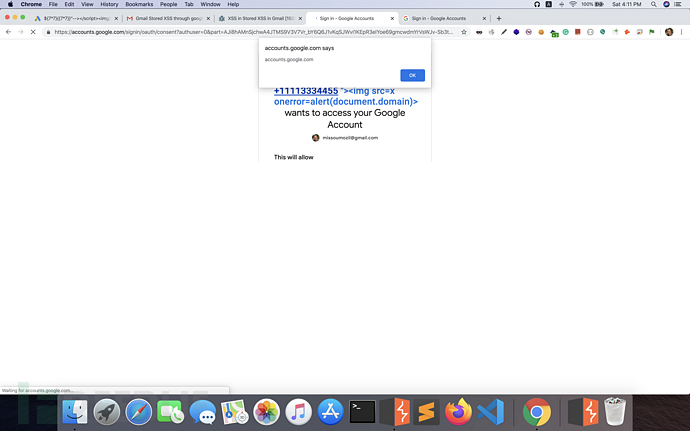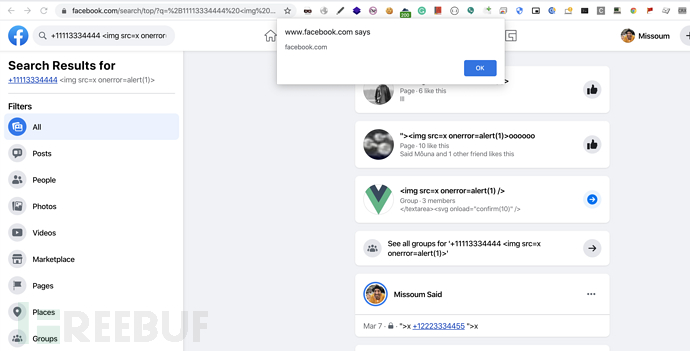## 漏洞分析于是乎，我把Google Voice的源代码提取出来进行了一番分析，果然在其中的文件contentscript.js中，存在一个方法函数Wg()，就是它导致了DOM XSS。该函数如下：

``````

function Wg(a) {

for (var b = /(^|\s)((\+1\d{10})|((\+1[ \.])?\(?\d{3}\)?[ \-\.\/]{1,3}\d{3}[ \-\.]{1,2}\d{4}))(\s|\$)/m, c = document.evaluate('.//text()[normalize-space(.) != ""]', a, null, XPathResult.UNORDERED_NODE_SNAPSHOT_TYPE, null), d = 0; d < c.snapshotLength; d++) {

a = c.snapshotItem(d);

var f = b.exec(a.textContent);

if (f && f.length) {

f = f;

var g = "gc-number-" + Ug,

h = '<span id="' + g + '" class="gc-cs-link"title="Call with Google Voice">' + f + "</span>",

k;

if (k = a.parentNode && !(a.parentNode.nodeName in Og)) k = a.parentNode.className,

k = "string" === typeof k && k.match(/\S+/g) || [], k = !Fa(k, "gc-cs-link");

if (k) try {

.snapshotLength) {

if (0 == a.parentNode.childElementCount) {

var w = a.parentNode.innerHTML,

y = w.replace(f, h);

a.parentNode.innerHTML = y

} else {

w = a.data;

y = w.replace(f, h);

var u = Qc("SPAN");

u.innerHTML = y;

h = u;

k = a;

v(null != h && null != k, "goog.dom.insertSiblingAfter expects non-null arguments");

k.parentNode && k.parentNode.insertBefore(h,

k.nextSibling);

Vc(a)

}

var t = Ic(document, g);

t && (Ug++, nc(t, "click", ma(Sg, t, f)))

}

} catch (E) {}

}

}

}

``````

``````

(var b = /(^|\s)((\+1\d{10})|((\+1[ \.])?\(?\d{3}\)?[ \-\.\/]{1,3}\d{3}[ \-\.]{1,2}\d{4}))(\s|\$)/m, c = document.evaluate('.//text()[normalize-space(.) != ""]', a, null, XPathResult.UNORDERED_NODE_SNAPSHOT_TYPE, null), d = 0; d < c.snapshotLength; d++) {

a = c.snapshotItem(d);

``````

2、如果已经抓取到了电话号码，就停止代码，防止继续遍历循环。

``````

w = a.parentNode.innerHTML,

y = w.replace(f, h);

a.parentNode.innerHTML = y

``````

``k.parentNode && k.parentNode.insertBefore(h, k.nextSibling);``

## 漏洞奖励

\$3,133.7

*参考来源：missoumsai，clouds 编译整理，转自 FreeBuf